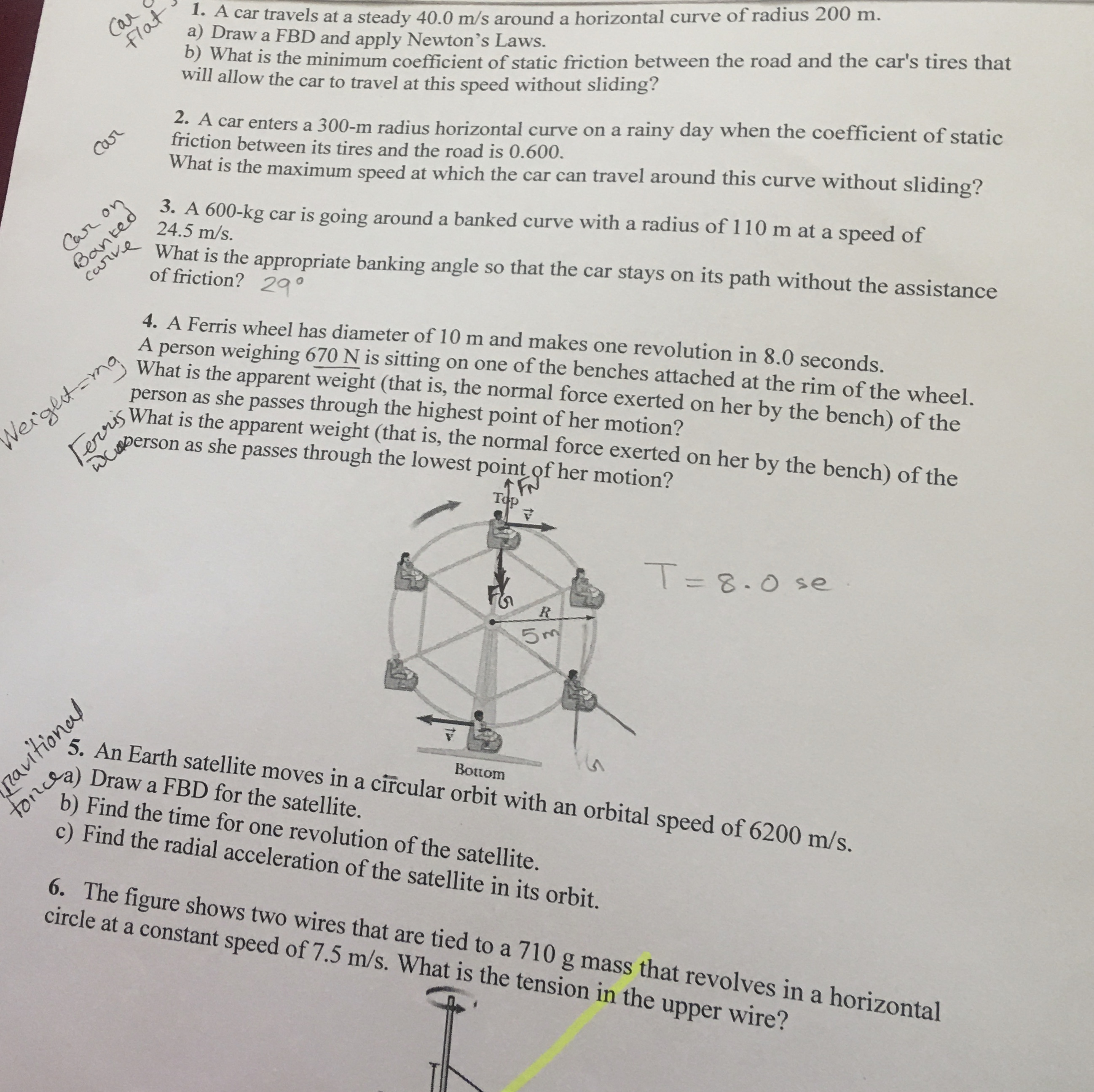# CarFlat1. A car travels at a steady 40.0 m/s arounda horizontal curve of radius 200 m.a) Draw a FBD and apply Newton's Laws.)What is the minimum coefficient of static friction between the road and the car's tires thatwill allow the car to travel at this speed without sliding?2. A car enters a 300-m radius horizontal curve on a rainy day when the coefficient of staticfriction between its tires and the road is 0.600.What is the maximum speed at which the car can travel around this curve without sliding?Carr3. A 600-kg car is going around a banked curve with a radius of 110 m at a speed of24.5 m/s.BantedcarveCar onWhat is the appropriate banking angle so that the car stays on its path without the assistanceof friction? 294. A Ferris wheel has diameter of 10 m and makes one revolution in 8.0 seconds.person weighing 670 N is sitting on one of the benches attached at the rim of the wheel.What is the apparent weight (that is, the normal force exerted on her by the bench) of theperson as she passes through the highest point of her motion?What is the apparent weight (that is, the normal force exerted on her by the bench) of theATerrisWberson as she passes through the lowest point of her motion?NergetmgTopT8.0 seR5 m5. An Earth satellite moves in a circular orbit with an orbital speed of 6200 m/s.toncgyb) Find the time for one revolution of the satellite.c) Find the radial acceleration of the satellite in its orbit.Draw a FBD for the satellite.TnBottom6. The figure shows two wires that are tied to a 710 g mass that revolves in acircle at a constant speed of 7.5 m/s. What is the tension in thehorizontalupper wire?autonal

Question

Number 3. I need also the free body diagram for that.help_outlineImage TranscriptioncloseCar Flat 1. A car travels at a steady 40.0 m/s arounda horizontal curve of radius 200 m. a) Draw a FBD and apply Newton's Laws. )What is the minimum coefficient of static friction between the road and the car's tires that will allow the car to travel at this speed without sliding? 2. A car enters a 300-m radius horizontal curve on a rainy day when the coefficient of static friction between its tires and the road is 0.600. What is the maximum speed at which the car can travel around this curve without sliding? Carr 3. A 600-kg car is going around a banked curve with a radius of 110 m at a speed of 24.5 m/s. Banted carve Car on What is the appropriate banking angle so that the car stays on its path without the assistance of friction? 29 4. A Ferris wheel has diameter of 10 m and makes one revolution in 8.0 seconds. person weighing 670 N is sitting on one of the benches attached at the rim of the wheel. What is the apparent weight (that is, the normal force exerted on her by the bench) of the person as she passes through the highest point of her motion? What is the apparent weight (that is, the normal force exerted on her by the bench) of the A Terris Wberson as she passes through the lowest point of her motion? Nergetmg Top T8.0 se R 5 m 5. An Earth satellite moves in a circular orbit with an orbital speed of 6200 m/s. toncgy b) Find the time for one revolution of the satellite. c) Find the radial acceleration of the satellite in its orbit. Draw a FBD for the satellite. Tn Bottom 6. The figure shows two wires that are tied to a 710 g mass that revolves in a circle at a constant speed of 7.5 m/s. What is the tension in the horizontal upper wire? autonal fullscreen
check_circleExpert Solution
Step 1

The free body diagram of the car is shown below.

Step 2

From the free body diagram, write the expression for ne...

### Want to see the full answer?

See Solution

#### Want to see this answer and more?

Solutions are written by subject experts who are available 24/7. Questions are typically answered within 1 hour*

See Solution
*Response times may vary by subject and question
Tagged in

### Physics